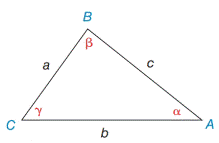Chapter 11.4, Problem 3E### Elementary Geometry for College St...

6th Edition
Daniel C. Alexander + 1 other
ISBN: 9781285195698

#### Solutions

Chapter
Section### Elementary Geometry for College St...

6th Edition
Daniel C. Alexander + 1 other
ISBN: 9781285195698
Textbook Problem
1 views

# In Exercises 3 and 4, state the form of the Law of Sines used to solve the problem. Give the answer in a form such as sin   72 ∘ 6.3 = sin   55 ∘ a a) Find β if it is known that a = 5 ,   b = 8 ,  and  α = 40 ∘ . b) Find c if it is known that a = 5.3   α = 41 ∘ ,  and  γ =87 ∘Exercises 1-8

To determine

a.

To Find:

The angle β using the Law of Sines.

Explanation

Formula:

The Law of Sines,

sinαa=sinβb=sinγc

Where α, β, and γ is the acute angle of the triangle, and a, b, and c is the sides of the triangle.

Calculation:

Given,

In ABC, a=5

b=8 and

α=40

To determine

b.

To Find:

The side c using the Law of Sines.

### Still sussing out bartleby?

Check out a sample textbook solution.

See a sample solution

#### The Solution to Your Study Problems

Bartleby provides explanations to thousands of textbook problems written by our experts, many with advanced degrees!

Get Started

#### Define sampling with replacement and explain why is it used?

Statistics for The Behavioral Sciences (MindTap Course List)

#### Find f(n)(x) if f(x) = 1/(2 x).

Single Variable Calculus: Early Transcendentals, Volume I

#### Graph each circle. x2+y216=0

College Algebra (MindTap Course List)